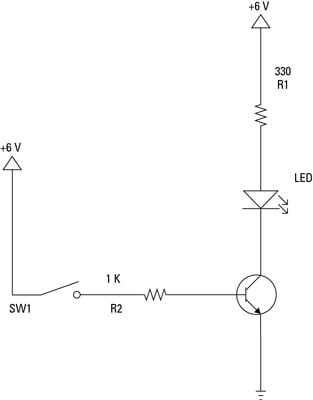##### Circuit Analysis For DummiesOne of the most common uses for transistors in an electronic circuit is as simple switches. In short, a transistor conducts current across the collector-emitter path only when a voltage is applied to the base. When no base voltage is present, the switch is off. When base voltage is present, the switch is on.

In an ideal switch, the transistor should be in only one of two states: off or on. The transistor is off when there's no bias voltage or when the bias voltage is less than 0.7 V. The switch is on when the base is saturated so that collector current can flow without restriction.

This is a schematic diagram for a circuit that uses an NPN transistor as a switch that turns an LED on or off.Look at this circuit component by component:

• LED: This is a standard 5 mm red LED. This type of LED has a voltage drop of 1.8 V and is rated at a maximum current of 20 mA.

• R1: This 330 Ω resistor limits the current through the LED to prevent the LED from burning out. You can use Ohm's law to calculate the amount of current that the resistor will allow to flow. Because the supply voltage is +6 V, and the LED drops 1.8 V, the voltage across R1 will be 4.2 V (6 – 1.8). Dividing the voltage by the resistance gives you the current in amperes, approximately 0.0127 A. Multiply by 1,000 to get the current in mA: 12.7 mA, well below the 20 mA limit.

• Q1: This is a common NPN transistor. A 2N2222A transistor was used here, but just about any NPN transistor will work. R1 and the LED are connected to the collector, and the emitter is connected to ground. When the transistor is turned on, current flows through the collector and emitter, thus lighting the LED. When the transistor is turned off, the transistor acts as an insulator, and the LED doesn't light.

• R2: This 1 kΩ resistor limits the current flowing into the base of the transistor. You can use Ohm's law to calculate the current at the base. Because the base-emitter junction drops about 0.7 V (the same as a diode), the voltage across R2 is 5.3 V. Dividing 5.3 by 1,000 gives the current at 0.0053 A, or 5.3 mA. Thus, the 12.7 mA collector current (ICE) is controlled by a 5.3 mA base current (IBE).

• SW1: This switch controls whether current is allowed to flow to the base. Closing this switch turns on the transistor, which causes current to flow through the LED. Thus, closing this switch turns on the LED even though the switch isn't placed directly within the LED circuit.

You might be wondering why you'd need or want to bother with a transistor in this circuit. After all, couldn't you just put the switch in the LED circuit and do away with the transistor and the second resistor? Of course you could, but that would defeat the principle that this circuit illustrates: that a transistor allows you to use a small current to control a much larger one.

If the entire purpose of the circuit is to turn an LED on or off, by all means omit the transistor and the extra resistor. But in more advanced circuits, you'll find plenty of cases when the output from one stage of a circuit is very small and you need that tiny amount of current to switch on a much larger current. In that case, this transistor circuit is just what you need.